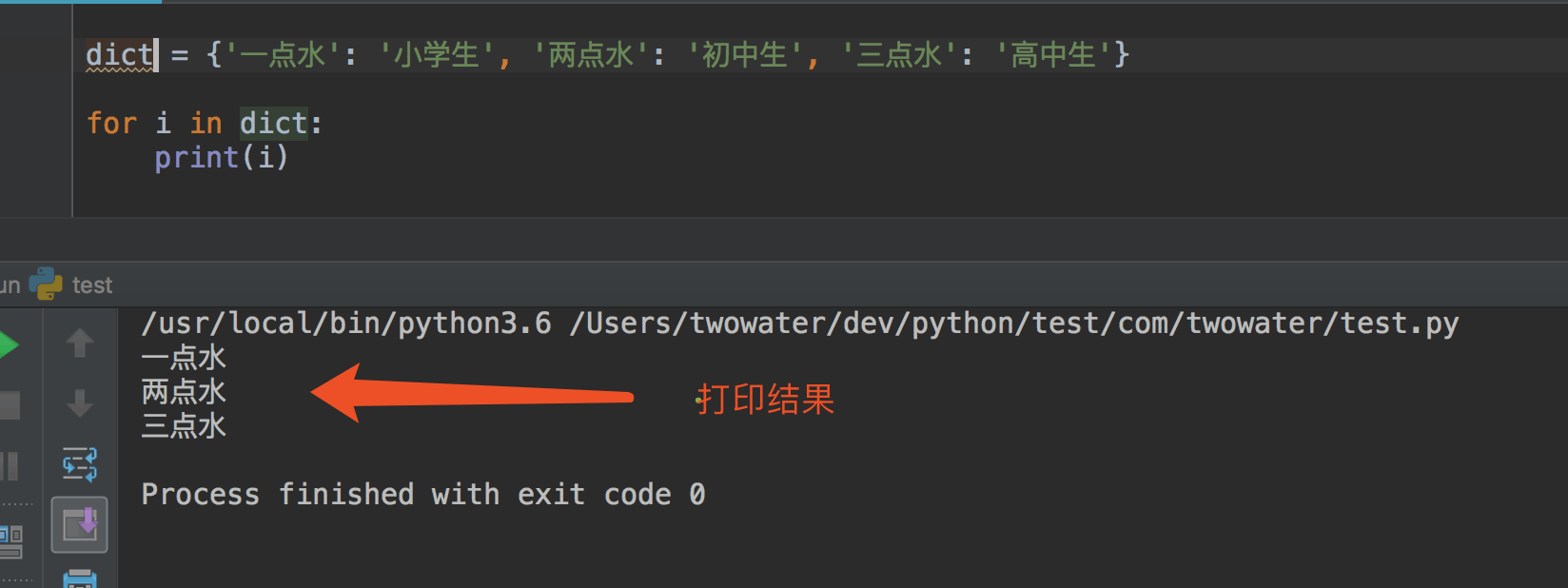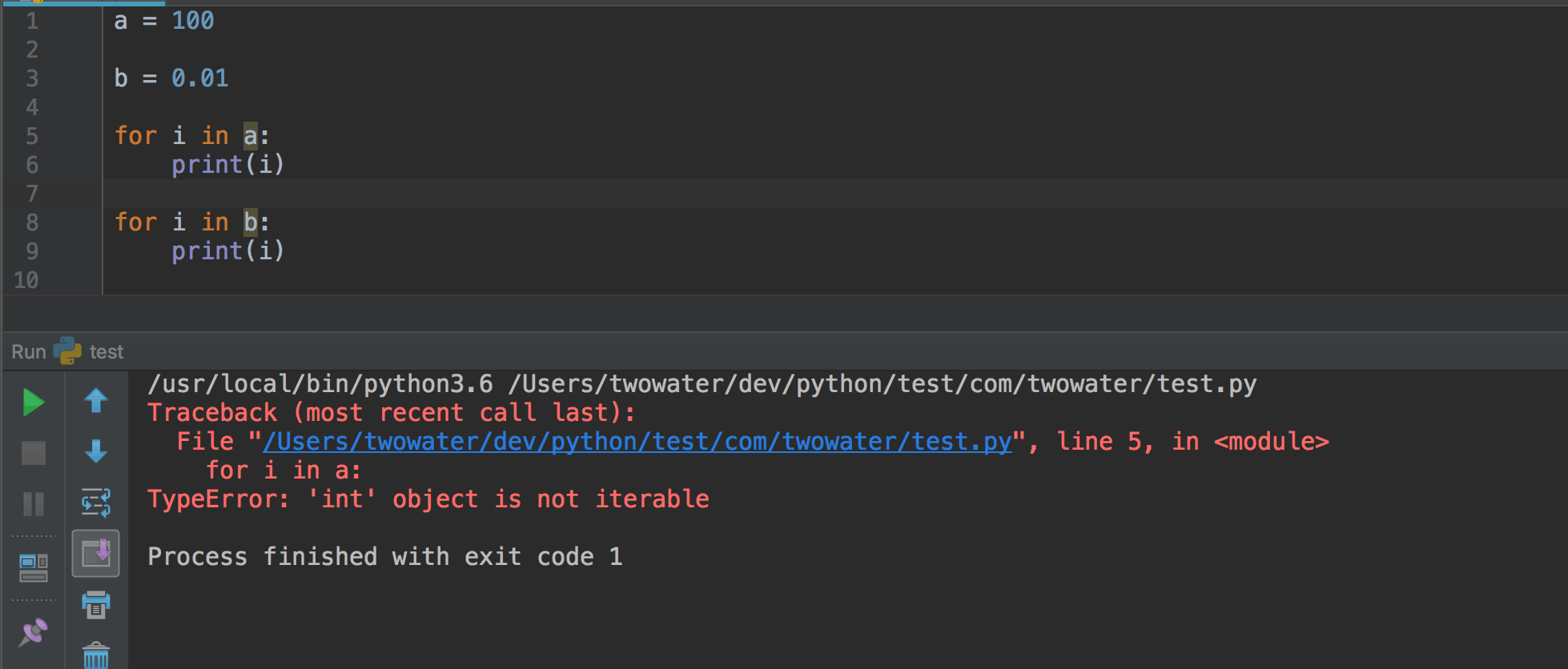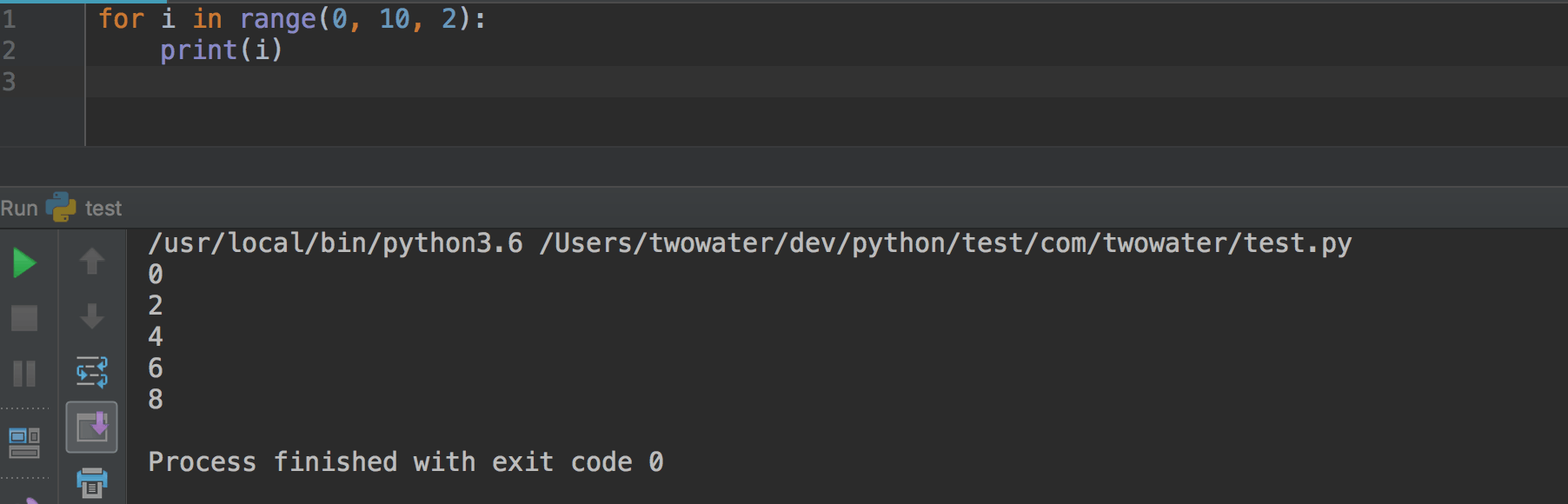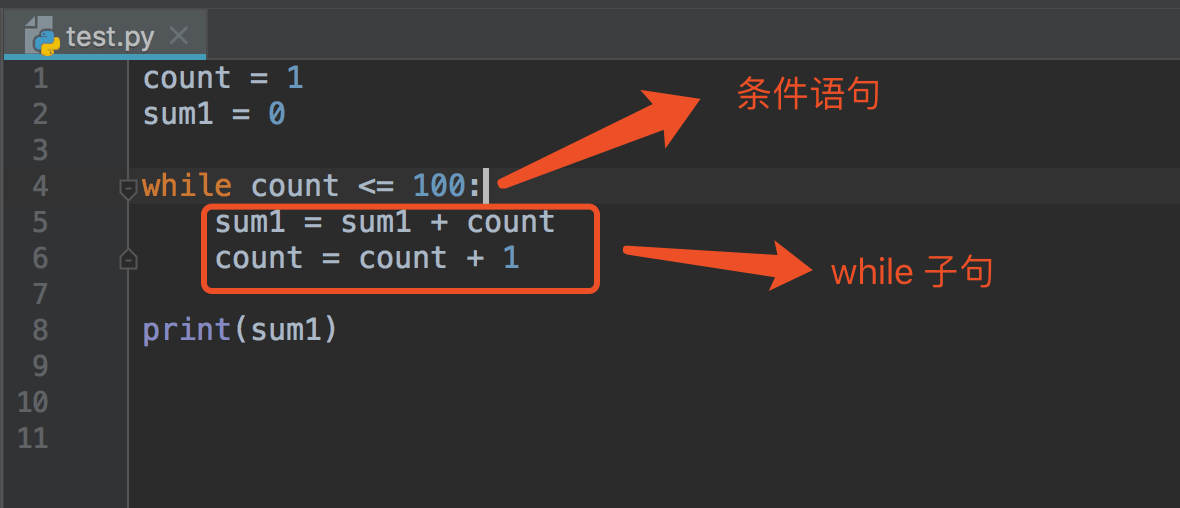# 二、循环语句

## 1、什么是循环语句break 在语句块执行过程中终止循环，并且跳出整个循环
continue 在语句块执行过程中终止当前循环，跳出该次循环，执行下一次循环
pass pass 是空语句，是为了保持程序结构的完整性

## 2、 for 循环语句``````for iterating_var in sequence:
statements(s)
``````

``````for letter in 'Hello 两点水':
print(letter)
``````

``````H
e
l
l
o

``````## 3、 range() 函数

for 循环还常常和 range() 函数搭配使用的。

``````for i in range(3):
print(i)
``````

``````0
1
2
``````

`range(3)` 就是 0 ，1，2 ，每次递增 1 。

`range(3,6)` 就是 3 ，4 ，5 ，也是每次递增 1 的。## 4、While 循环语句

While 循环和 for 循环的作用是一样的。``````5050
``````

## 5、for 循环和 whlie 循环的区别

• for 循环主要用在迭代可迭代对象的情况。

• while 循环主要用在需要满足一定条件为真，反复执行的情况。 （死循环+break 退出等情况。）

• 部分情况下，for 循环和 while 循环可以互换使用。

``````for i in range(0, 10):
print(i)

i = 0
while i < 10:
print(i)
i = i + 1
``````

## 6、嵌套循环

for 循环嵌套语法

``````for iterating_var in sequence:
for iterating_var in sequence:
statements(s)
statements(s)
``````

while 循环嵌套语法

``````while expression:
while expression:
statement(s)
statement(s)
``````

``````count = 1
sum = 0
while (count <= 100):
sum = sum + count
if ( sum > 1000):  #当 sum 大于 1000 的时候退出循环
break
count = count + 1
print(sum)
``````

``````1035
``````

``````count = 1
sum = 0
while (count <= 100):
if ( count % 2 == 0):  # 双数时跳过输出
count = count + 1
continue
sum = sum + count
count = count + 1
print(sum)
``````

``````2500
``````

``````for num in range(10,20):  # 迭代 10 到 20 之间的数字
for i in range(2,num): # 根据因子迭代
if num%i == 0:      # 确定第一个因子
j=num/i          # 计算第二个因子
print ('%d 是一个合数' % num)
break            # 跳出当前循环
else:                  # 循环的 else 部分
print ('%d 是一个质数' % num)
``````

``````10 是一个合数
11 是一个质数
12 是一个合数
13 是一个质数
14 是一个合数
15 是一个合数
16 是一个合数
17 是一个质数
18 是一个合数
19 是一个质数
``````